One of the problems that are given in mathematics is proof. You have to prove that the figures of triangles are equal. In this case, parallelograms are often used in proofs.

You will almost never be asked to prove that a shape is a parallelogram. On the other hand, problems that require you to prove that each triangle is congruent using the properties of parallelograms are frequent.

To solve proofs, you need to understand the properties of parallelograms. So, what is the definition of a parallelogram? And what are the theorems derived from the definition of a parallelogram?

After learning about the properties of parallelograms, we will explain how to solve the proof problems.

## Definition and Four Properties of Parallelograms

First of all, what kind of shape is a parallelogram? The definition of a parallelogram is as follows.

• A quadrilateral with two pairs of opposite sides that are parallel to each other.

In a parallelogram, the sides of a quadrilateral that face each other are called opposite sides. In a parallelogram, the angles facing each other in a quadrilateral are called opposite angles. The opposite sides and opposite angles are as follows.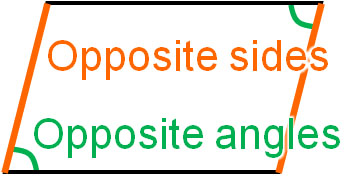In a parallelogram, the opposite sides are each parallel. In that case, we have the following properties.

• The opposite sides of the two pairs are equal in length.
• The opposite angles of the two pairs are equal.
• The consecutive angles add up to 180°.
• Two diagonals intersect at their midpoints.

Let’s review the properties of each.

### Two Pairs of Opposite Sides Are Equal in Length

If two pairs of opposite sides are parallel to each other, the lengths of the opposite sides will always be the same. Understand that the length of each side will be the same as shown below.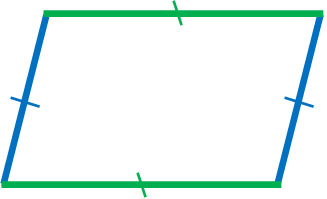When a parallelogram is presented in a problem, try to solve the problem using the property that the lengths of the opposite sides are equal. For example, if the length of one side of a parallelogram is 10 cm, the length of the opposite side is 10 cm.

### Two Pairs of Opposite Angles Are Equal to Each Other

In a parallelogram, the opposite angles are the same. This property is frequently used to calculate angles and to prove the congruence (or similarity) of triangles.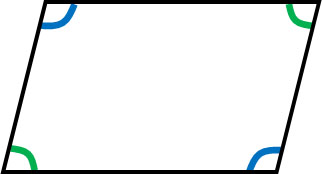Remember that the angles facing each other are always equal. For example, if one angle is 60°, the opposite angle is also 60°.

#### Proof That Opposite Sides and Angles Are Equal

So, is it really true that in a parallelogram, the opposite sides and the opposite angles are equal? Let’s try to prove this. To prove it, draw a diagonal as shown below.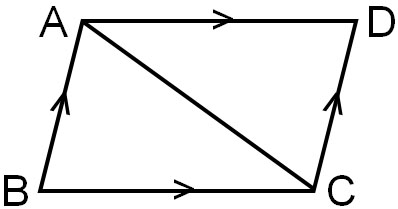From the definition of a parallelogram, AB||DC and AD||BC. In this case, let’s consider △ABC and △CDA. The result is as follows.

• In △ABC and △CDA
• AC = CA: common line – (1)
• ∠BAC = ∠DCA: Parallel lines have equal alternate angles – (2)
• ∠ACB = ∠CAD: Parallel lines have equal alternate angles – (3)
• From (1), (2), and (3), since Angle – Side – Angle (ASA), △ABC≅△CDA

Since △ABC≅△CDA, AB=DC and AD=BC. In other words, the opposite sides are equal.

Also, ∠B = ∠D, so the opposite angles are equal. For the other opposite angles, we can prove that the angles are equal by drawing another diagonal and proving that the triangles are congruent. In any case, in a parallelogram, the opposite angles are always equal.

### Consecutive Angles Add UP to 180°

Since the opposite angles are equal and the lines are parallel to each other, parallelograms have other properties. A theorem of parallelograms is that consecutive angles add up to 180°.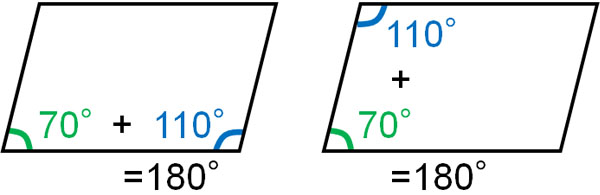In parallelogram problems, we frequently use this theorem to solve problems.

Why do consecutive angles add up to 180°? In a parallelogram, the opposite sides are parallel. And in parallel lines, the corresponding angles have the same angle. Therefore, we have the following.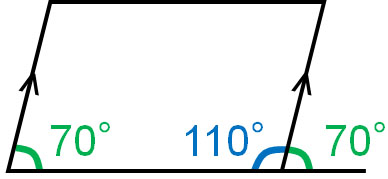In a parallelogram, if you know one angle, you can calculate all the other angles. We have already explained that the opposite angles are equal. The other angle can be calculated by subtracting the known angle from 180°.

### Two Diagonals Intersect at Their Midpoints

There is another theorem in parallelograms. In a parallelogram, we can draw two diagonals, and the intersection of the diagonals is the midpoint. It is as follows.Since it is a midpoint, it creates two lines of the same length. We often have to use this property to solve geometry problems.

#### Proof That Diagonals Intersect at the Midpoint

Why do the diagonals in a parallelogram intersect at the midpoint? Let’s explain the reason with proof. Consider the following parallelogram.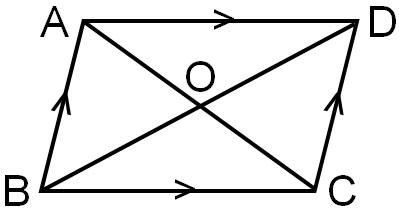For △AOB and △COD, prove that they are congruent. Then we can explain that the intersection of the diagonals is the midpoint.

• In △AOB and △COD
• AB = DC: The opposite sides of a parallelogram are equal – (1)
• ∠BAO = ∠DCO: The alternate angles in parallel lines are equal – (2)
• ∠ABO = ∠CDO: The alternate angles in parallel lines are equal – (3)
• From (1), (2), and (3), since Angle – Side – Angle (ASA), △AOB≅△COD

Since △AOB≅△COD, AO=CO and BO=DO. Therefore, we know that in a parallelogram, the intersection of the diagonals is the midpoint.

## When All Four Angles Are Equal, It Becomes a Rectangle

There are some special shapes among parallelograms. One of them is a rectangle. If certain conditions are met, a parallelogram becomes a rectangle. As mentioned above, parallelograms have equal opposite angles. If all the angles are equal, it is a rectangle.

In order for all angles to be equal, the angles must be 90°. Although the side lengths can be different, if all the angles are equal, then the following is true.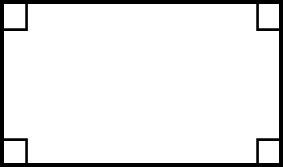A rectangle has parallel opposite sides and is a type of parallelogram. However, a parallelogram in which all angles are equal is called a rectangle.

### If All Four Sides Are Equal, It’s a Rhombus

There is another special kind of parallelogram. It is a rhombus. In a rhombus, all sides are equal in length.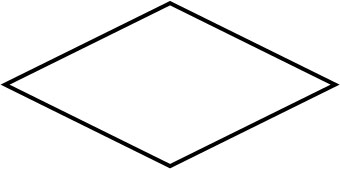In a rectangle, all angles are equal. In a rhombus, on the other hand, all the sides are equal in length, but not all the angles need to be equal.

The definition of a rhombus is all four sides are equal in length. A theorem derived from this definition is the diagonals intersect perpendicularly. It is as follows.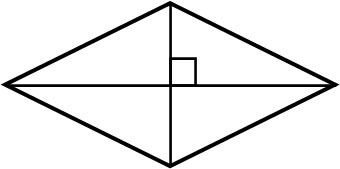By drawing diagonals on a rhombus, we can create right triangles.

-A square Has Properties of a Rectangle and a Rhombus

What about a square that has all the same angles and side lengths? In this case, it will have the properties of both a rectangle and a rhombus. Of course, the diagonals intersect perpendicularly.

## Exercise: Proof of Congruence

Q1: Prove the following figure.

There is a parallelogram ABCD. Let O be the intersection point of the diagonals, and prove that △AOE≅△COF when OE=OF.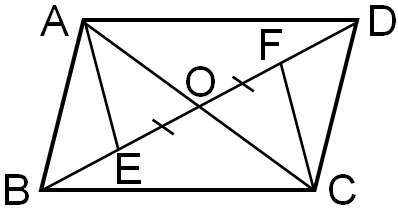Why is it important to understand the properties of parallelograms? It is because without understanding the definition and theorem of parallelograms, you will not be able to solve mathematical problems. Learn the properties of parallelograms so that you can solve the problems.

By using the definitions and theorems of parallelograms, we can prove the following.

• In △AOE and △COF
• OE = OF: Given – (1)
• ∠AOE = ∠COF: Vertical angles are equal – (2)
• AO=CO: Diagonals in a parallelogram intersect at the midpoint – (3)
• From (1), (2), and (3), since Side – Angle – Side (SAS), △AOE≅△COF

In geometry problems that use parallelograms, there is a high probability that you will use the parallelogram theorem to solve the problem. Be sure to memorize the properties of parallelograms because you will not be able to solve them unless you remember them.

## Using Properties of Parallelograms to Solve Proof Problems

There are many times in mathematics when you have to solve problems involving figures. So, you need to understand the properties of parallelograms.

As with all geometry problems, you need to remember what properties the shape has in order to solve the problem. Therefore, it is important to remember not only the properties of triangles such as isosceles triangles and right triangles but also parallelograms.

A parallelogram is a shape in which two pairs of opposite sides are parallel. There are four main properties that can be derived from this definition. By using these theorems, we can solve problems involving shapes.

In particular, the properties of parallelograms are frequently used in proofs of figures. Let’s prove the congruence (or similarity) of triangles using the properties of parallelograms.